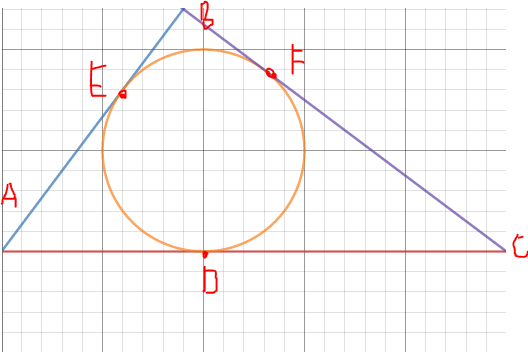# What, again?

Geometry Level 3In the above figure, $AB=3$, $BC = 4$ and $AC = 5$. Find $AD \times DC$.

×